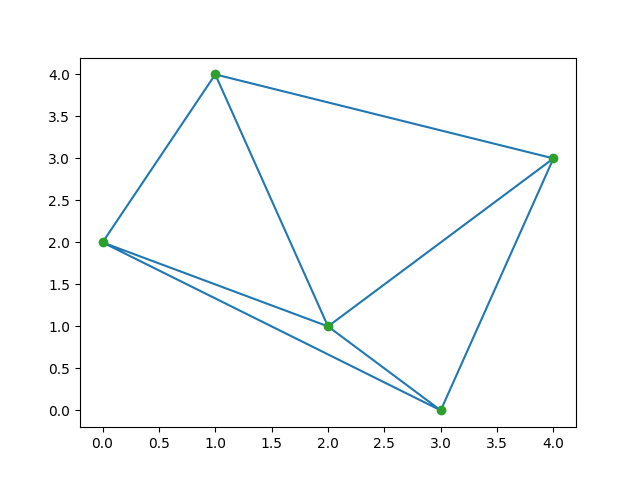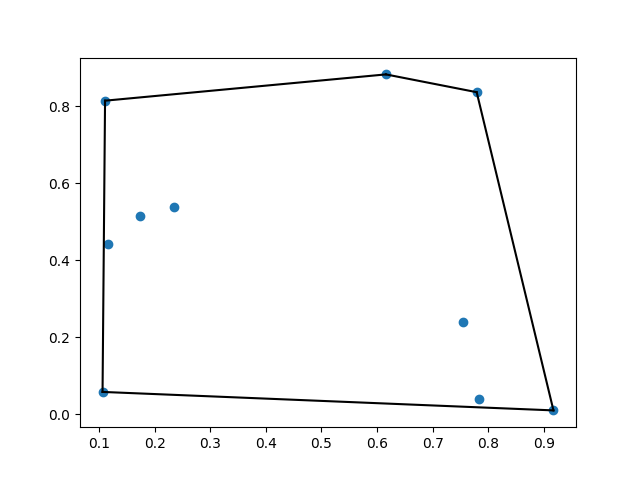# scipy.spatial – Spatial data structures and algorithms

• Last Updated : 17 Oct, 2021

In this article, we are going to see spatial data structure and algorithms, it is used to represent data in a geometric space.

## What is spatial data structure?

The spatial package computes the triangulations, Voronoi diagrams, and convex hulls of a set of points, by leveraging the Qhull library. Moreover, it contains KDTree implementations for nearest neighbor point queries and utilities for distance computations in various metrics.

Attention geek! Strengthen your foundations with the Python Programming Foundation Course and learn the basics.

To begin with, your interview preparations Enhance your Data Structures concepts with the Python DS Course. And to begin with your Machine Learning Journey, join the Machine Learning - Basic Level Course

### Example 1: Delaunay Triangulations

In mathematics and computational geometry, a Delaunay triangulation for a given set p of discrete points, in a plane is a triangulation DT(p) such that no point p is inside the circumcircle of any triangle in DT(p).

## Python

 `from` `scipy.spatial ``import` `Delaunay ` `import` `numpy as np ` `import` `matplotlib.pyplot as plt ` ` `  `points ``=` `np.array([[``1``, ``4``], [``2``, ``1``], [``3``, ``0``],  ` `                   ``[``0``, ``2``], [``4``, ``3``]]) ` `tri ``=` `Delaunay(points) ` ` `  `plt.triplot(points[:, ``0``], points[:, ``1``], tri.simplices.copy()) ` `plt.plot(points[:, ``0``], points[:, ``1``], ``'o'``) ` `plt.show() `

Output:### Example 2: Coplanar points

Coplanar points are three or more points that lie in the same plane. Recall that, a plane is a flat surface, which extends without end in all directions.

## Python

 `from` `scipy.spatial ``import` `Delaunay ` `import` `numpy as np ` ` `  `points ``=` `np.array([[``0``, ``0``], [``0``, ``1``], [``1``, ``0``],  ` `                   ``[``1``, ``1``], [``1``, ``1``]]) ` `tri ``=` `Delaunay(points) ` `print``(tri.simplices) ` `print``(tri.coplanar) `

Output:

```[[3 1 0]
[2 3 0]]
[[4 0 3]]```

### Example 3: Convex Hulls

The convex hull or convex envelope of a set of points X in the euclidean space(or, more generally in affine space over the reals) is the smallest convex set that contains X.

## Python

 `from` `scipy.spatial ``import` `ConvexHull ` `import` `numpy as np ` `import` `matplotlib.pyplot as plt ` ` `  `points ``=` `np.random.rand(``10``, ``2``) ` `hull ``=` `ConvexHull(points) ` ` `  `plt.plot(points[:, ``0``], points[:, ``1``], ``'o'``) ` `for` `simplex ``in` `hull.simplices: ` `    ``plt.plot(points[simplex, ``0``], points[simplex, ``1``], ``'k-'``) ` ` `  `plt.show() `

Output:### Example 4: KPTrees

kd-tree is a quick nearest-neighbor lookup. And Kdtree() methods return the kd-tree object

## Python3

 `from` `scipy.spatial ``import` `KDTree ` ` `  `points ``=` `np.random.rand(``10``, ``2``) ` `kdtree ``=` `KDTree(points) ` `result ``=` `kdtree.query((``1``, ``1``)) ` `print``(result) `

Output:

`(0.5144859720297681, 9)`

My Personal Notes arrow_drop_up
Recommended Articles
Page :